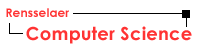CSCI 4972/6963 - Spring 2008
Home
Contact Information
Office Hours

Syllabus
Prerequisites
Textbook

Calendar
Lecture notes
Lab materials
Homework
Test reviews

Homework
Collaboration Policy
Compilers
gcc/g++ notes
GL/glut notes
Homework Late Policy
Electronic Submission

# Homework 0: Transformations & OpenGL Warmup

The goal of this warmup assignment is to get comfortable with the programming environment you will be using for this class and familiarize yourself with a simple library that we will use for linear algebra. This assignment will not be formally graded, but you are expected to work through and submit the exercises. It's also an opportunity for a crash course in C++ and OpenGL (if you're not already familiar with them). Here are a couple tutorials you may want to check out: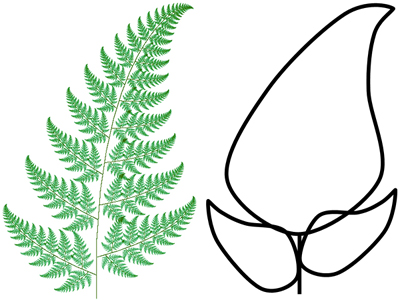The incidental goal is also to have fun with bizarre fractal objects. IFS are self-similar fractals: a subpart of the object is similar to the whole. The classic example of an IFS is Barnsley's fern, where each subpart of the fern is exactly the same as the whole fern. IFS are described by a set of affine transformations (rotations, translations, scale, skew, etc.) These transformations capture the self-similarity of the object. IFS can be defined in any dimension, and we will play with both two-dimensional and three-dimensional ones. Formally, an IFS is defined by n affine transformations. Each transformation fi must be contractive: The distance between points must be reduced. An attractor of the IFS is the object such that A = U fi (A). A is unchanged by the set of transformations: It is a fixed point.

We can render an IFS by iterating the transform on random input points from the unit square. We approximate the fixed point by applying the transformation many times. The algorithm is as follows:

```   for "lots" of random points (x0, y0)
for k=0 to num_iters
pick a random transform fi
(xk+1, yk+1) = fi(xk, yk)
display a dot at (xk, yk)
```

To reduce the number of points necessary to make an image of reasonable quality, probabilities are assigned to each transformation, instead of choosing a transformation with uniform probability.

• Download the provided source code and set up your C++ development environment. To receive full credit, your homework assignments must compile and run without errors using gcc/g++. Even if you plan to do much of your development in another environment, you'll probably still want to set up gcc so that you can test it before submission. For interactive display of your IFS, you will use the OpenGL API that uses graphics hardware for fast rendering of 3D primitives. Note: with some configurations, software emulation might be used, resulting in slower rendering. Here are some notes:

A Makefile is provided for use with either Unix or Cygwin. On Linux or FreeBSD, type: make unix . On Windows/Cygwin, if you have the files OpenGL32.lib, GLU32.lib, and glut32.lib, type: make cygwin_lib ; if you have libopengl32.a, libglu32.lib, and libglut32.a, type: make cygwin_a ; and if you have libopengl.a, libglu.a, and libglut.a, type: make cygwin_x . On Mac OSX, type: make osx . You may need to adjust the CC, INCLUDE_PATH, and LIB_PATH lines of the Makefile for your particular installation to point to the right files. Please see the TA if you have trouble setting up your development environment.

All files implementing OpenGL code should include the OpenGL header files:

```   #include <GL/gl.h>
#include <GL/glu.h>
#include <GL/glut.h>
```

When provided with appropriate arguments, the initial executable should launch an OpenGL window and draw a cube and you should be able to navigate the scene with the mouse (left button rotates, middle button translates, right button zooms). Try this at the command prompt:

```   ifs -size 500
```

• Now you're ready to start coding. Write a C++ class IFS that renders iterated function systems, including the class declaration (in the header file ifs.h) and the implementation (ifs.C). The IFS class should include:
• a field to store n, the number of transformations,
• an array/vector of matrices representing the n transformations,
• an array/vector of the corresponding probabilities for choosing a transformation,
• a function that parses the input file and creates an IFS,
• a paint method which makes appropriate OpenGL calls to draw points or polygons to the OpenGL window, and

• When you're done, adjust the main function (provided in main.C) as necessary.

• Use the linear algebra library for the point and transformation representations.

• Consider the performance of your programming environment. How many polygons/points can you render interactively? What improvements could you make to your code?## Possible Extensions

• design a new IFS --- figure out the transformations and probabilities,
• automatically determine the bounding box of the fractal object,
• change the color scheme,
• implement anti-aliasing,
• experiment with depth-first vs. breadth-first, etc.

## Hints

• Random numbers can be obtained using the drand48() or rand() and RAND_MAX. See stdlib.h.
• To debug your code, set the number of iterations to one. This will allow you to check that you got the transformations right.
• Be careful, arrays are indexed from 0 to n-1 in C++. Reading beyond the bounds of the array will probably result in a segmentation fault.
• Use assert() to check function pre-conditions, array indices, etc. See assert.h.
• To perform transformations in OpenGL, read about the Modelview matrix stack and the OpenGL commands glMatrixMode(), glPushMatrix(), glPopMatrix(), and glMultMatrix() in the OpenGL Programming Guide, Chapter 3.

## Provided Files (hw0_files.zip, updated 1/16@1pm)

• Linear Algebra Library (vectors.h & matrix.h & matrix.cpp)
Linear algebra support for floating point vectors with 2, 3, and 4 elements (Vec2f, Vec3f, and Vec4f) and 4x4 floating point matrices (Matrix). For this assignment, the void Matrix::Transform(Vec3f &v) function will be handy.
• Parsing code for command-line arguments and input files (argparser.h & parse.cpp)
Your program should take a number of command line arguments to specify the input file (-input), number of points (-points), number of iterations (-iters), and output size (-size). Your program should render points by default, or cubes if -cubes is specified. Examples are shown below. Code to parse input files and command line arguments is provided.
• OpenGL and main code (main.cpp, ifs.h, glCanvas.h, glCanvas.cpp, camera.h, camera.cpp)
OpenGL programs can be tricky to set up from scratch. This base code should do all that work for you.
• Makefile for g++ on UNIX or Windows/Cygwin
On the command line simply type make unix (or make cygwin_lib or make cygwin_a or make cygwin_x or make osx). To force a re-build of all object files, type make clean first.
• Data files (fern.txt, dragon.txt, sierpinski_triangle.txt, and giant_x.txt)
The input data for an IFS is a file which contains n, the number of transforms, followed by the probability of choosing each transform and a 4x4 floating point matrix representation of the transform.

## Sample Results

```ifs -input sierpinski_triangle.txt -points 10000 -iters 0 -size 200
ifs -input sierpinski_triangle.txt -points 10000 -iters 1 -size 200
ifs -input sierpinski_triangle.txt -points 10000 -iters 2 -size 200
ifs -input sierpinski_triangle.txt -points 10000 -iters 3 -size 200
ifs -input sierpinski_triangle.txt -points 10000 -iters 4 -size 200
ifs -input sierpinski_triangle.txt -points 10000 -iters 30 -size 200

```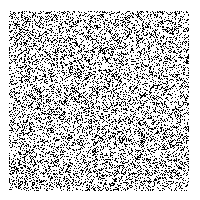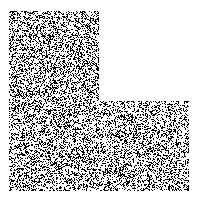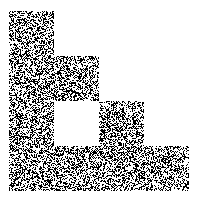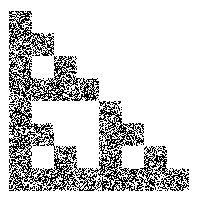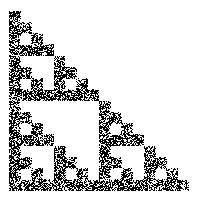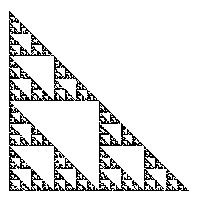```ifs -input fern.txt -points 50000 -iters 30 -size 400
``````ifs -input giant_x.txt -points 10000 -size 400 -iters 0
ifs -input giant_x.txt -points 10000 -size 400 -iters 1
ifs -input giant_x.txt -points 10000 -size 400 -iters 2
ifs -input giant_x.txt -points 10000 -size 400 -iters 3
ifs -input giant_x.txt -points 10000 -size 400 -iters 4
```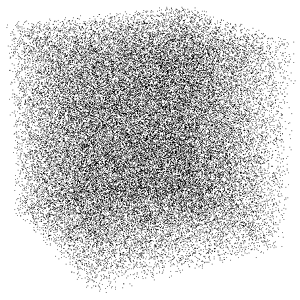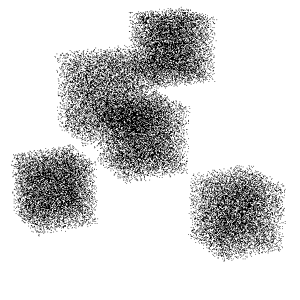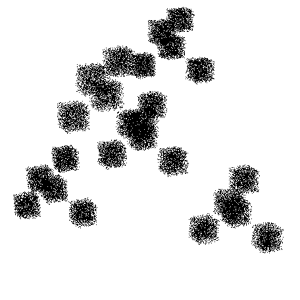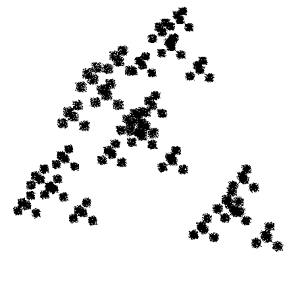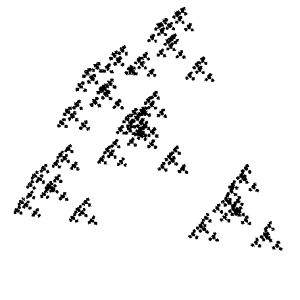```ifs -input giant_x.txt -size 400 -iters 0 -cubes
ifs -input giant_x.txt -size 400 -iters 1 -cubes
ifs -input giant_x.txt -size 400 -iters 2 -cubes
ifs -input giant_x.txt -size 400 -iters 3 -cubes
ifs -input giant_x.txt -size 400 -iters 4 -cubes
```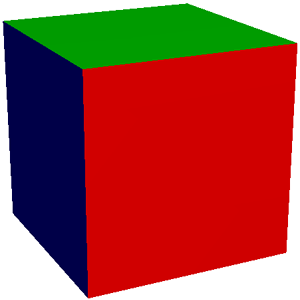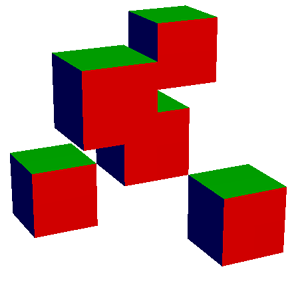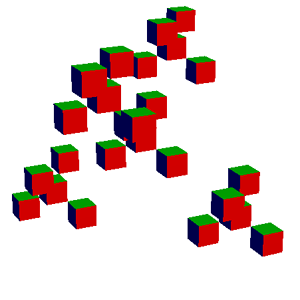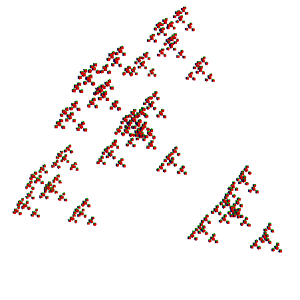Please read the Homework information page again before submitting. Also, note that this assignment will not be graded.